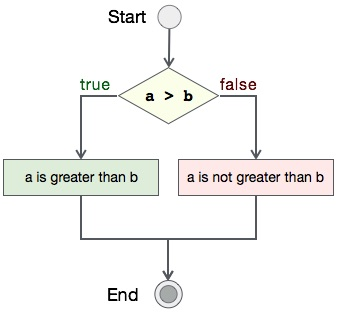# Program to delete a file in C

## Algorithm

Let's first see what should be the step-by-step procedure to compare two integers−

```START

Step 1 → Take two integer variables, say A & B

Step 2 → Assign values to variables

Step 3 → Compare variables if A is greater than B

Step 4 → If true print A is greater than B

Step 5 → If false print A is not greater than B

STOP
```

## Flow Diagram

We can draw a flow diagram for this program as given below −## Pseudocode

Let's now see the pseudocode of this algorithm −

```procedure compare(A, B)

IF A is greater than B
DISPLAY "A is greater than B"
ELSE
DISPLAY "A is not greater than B"
END IF

end procedure
```

## Implementation

Assuming we have file with name file_append.txt with this content −

```This text was already there in the file.
```

Now, we shall see the actual implementation of the program −

```#include <stdio.h>
#include <string.h>

int main ()
{
int ret;
FILE *fp;
char filename[] = "file.txt";

fp = fopen(filename, "w");

fprintf(fp, "%s", "This is tutorialspoint.com");
fclose(fp);

ret = remove(filename);

if(ret == 0)
{
printf("File deleted successfully");
}
else
{
printf("Error: unable to delete the file");
}

return(0);
}
}
```

## Output

Output of this program should be −

```File deleted successfully
```